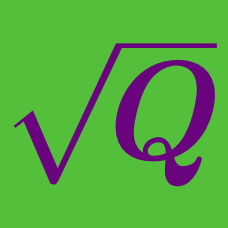Algebra

# Radical Expressions and Equations: Level 1 Challenges

$\Large 2^{\color{#D61F06}3} + 2^{\color{#D61F06}3} + 3^{\color{#3D99F6}4} + 3^{\color{#3D99F6}4} + 3^{\color{#3D99F6}4} = {\ \color{teal}} ?$

If half of $\sqrt{2}$ is $\sqrt{k}$, then what is the value of $k?$

$\large \sqrt{\frac{1}{\sqrt{ x}}}=3, \ \ \ x = \ ?$

$\large \frac{\sqrt{12}-\sqrt{27}}{6}\times\sqrt{3}\times\sqrt{4}= \ ?$

$\large\color{#20A900} 6^{\color{#3D99F6} 6} + \color{#20A900} 6^{\color{#3D99F6} 6} + \color{#20A900} 6^{\color{#3D99F6} 6} + \color{#20A900} 6^{\color{#3D99F6} 6} + \color{#20A900} 6^{\color{#3D99F6} 6} + \color{#20A900} 6^{\color{#3D99F6} 6} = \color{#20A900}6^ {\color{#624F41}a}$

If $\color{#624F41} a$ satisfies the equation above, what is the value of $\color{#624F41} a$?

×